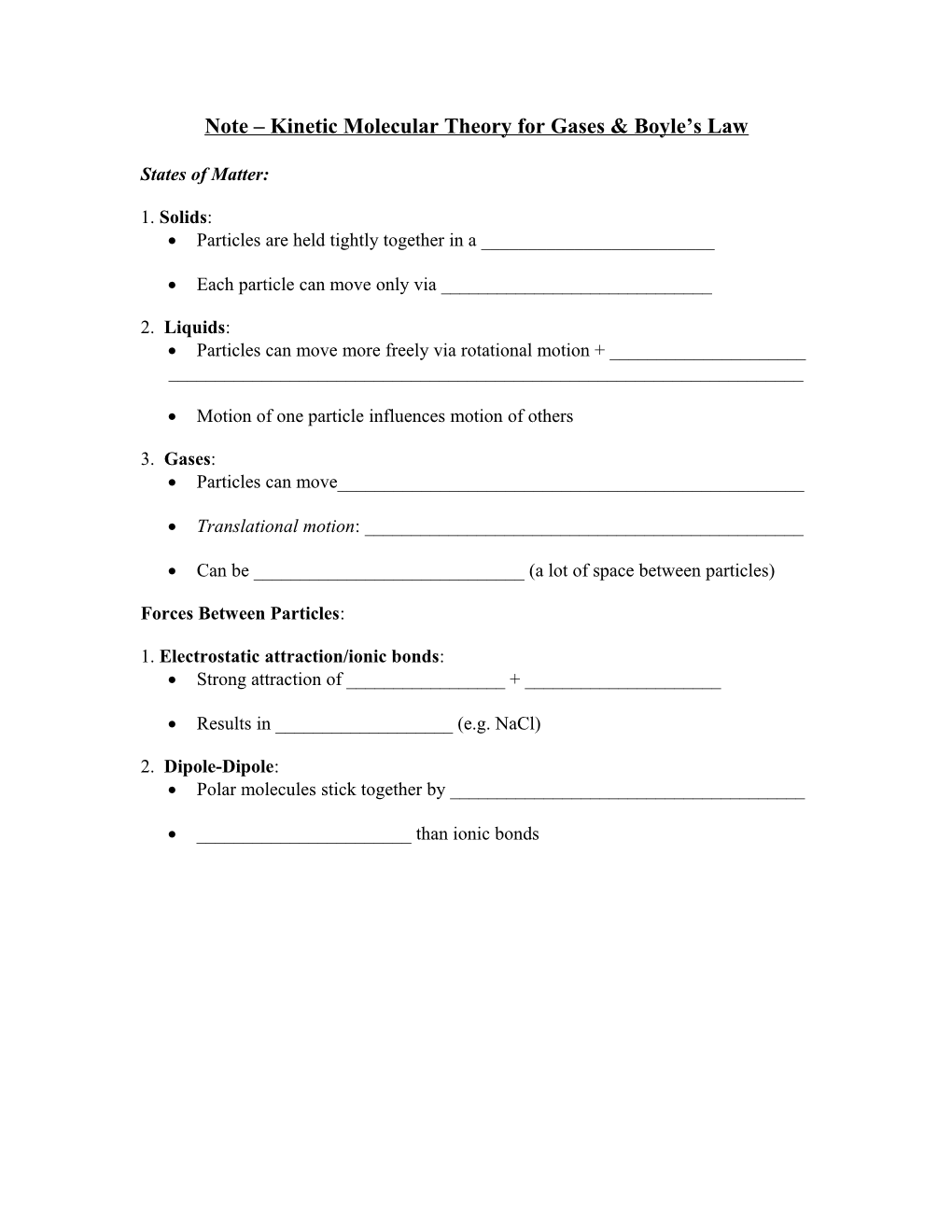# Note Kinetic Molecular Theory for Gases & Boyle S LawNote – Kinetic Molecular Theory for Gases & Boyle’s Law

States of Matter:

1. Solids:

• Particles are held tightly together in a ______
• Each particle can move only via ______

2. Liquids:

• Particles can move more freely via rotational motion + ______

______

• Motion of one particle influences motion of others

3. Gases:

• Particles can move______
• Translational motion: ______
• Can be ______(a lot of space between particles)

Forces Between Particles:

1. Electrostatic attraction/ionic bonds:

• Strong attraction of ______+ ______
• Results in ______(e.g. NaCl)

2. Dipole-Dipole:

• Polar molecules stick together by ______
• ______than ionic bonds

3. London Dispersion Forces:

• E.g H2
• Small non-polar molecule:

- Fewer sites of temporary dipoles

-

• Large non-polar molecule:

-Many temporary dipoles can exist

-Molecules can ______

Heating Curve of Water:

Kinetic Molecular Theory (KMT) for Gases:4 Assumptions:

1. Volume of individual particle is Zero.
1. Particles are inconstant motion. Collision of particles against container walls is the cause of pressure.
1. Particles exert no forces on each other (neither attract nor repel)
1. Average speed of particles is proportional to temperature in Kelvins.

Gas Relationships explained using KMT:

1. Pressure and Volume:
2. How does pressure change if volume is decreased?

Pressure:

-SI Unit =

Other units:

Boyle’s Law:

-If the volume of a gas increases, ______

-If the volume of a gas decreases, ______

Sample 1: Consider a sample of sulfur dioxide gas that is in a 1.53 L container at a pressure of 5.6 x 103 Pa. If the pressure is changed to 1.5 x 104 Pa at a constant temperature what will be the new volume?

Sample 2: A diver at a depth of 100m experiences 9.7atm of pressure. At this depth his lung volume is 6.0 L. to what volume would his lungs expand if he were immediately brought to the surface (P = 1.0 atm, temperature stays constant)?

BOYLE’S LAW PROBLEMS

Abbreviations Conversions

atm atmosphere K = C + 273

mm Hg millimeters of mercury 1 cm3 (cubic centimeter) = 1 mL (milliliter)

torr another name for mm Hg 1 dm3 (cubic decimeter)=1 L(liter)=1000 mL

Pa Pascal (kPa = kilo Pascal) Standard Conditions

K Kelvin 0.00 C = 273 K

C degrees Celsius 1.00 atm = 760.0 mm Hg =101.325 kPa = 760.0 torr

1. A gas occupies 12.3 liters at a pressure of 40.0 mm Hg. What is the volume when the

pressure is increased to 60.0 mm Hg?

2. If a gas at 25.0 C occupies 3.60 liters at a pressure of 1.00 atm, what will be its volume at a pressure of 2.50 atm?

3. To what pressure must a gas be compressed in order to get into a 3.00 cubic foot tank the entire weight of a gas that occupies 400.0 cu. ft. at standard pressure?

4. If the pressure on a gas is decreased by onehalf, how large will the volume change be?

5. A gas occupies 4.31 liters at a pressure of 0.755 atm. Determine the volume if the pressure is increased to 1.25 atm.

6. A sample of gas has a volume of 12.0 L and a pressure of 1.00 atm. If the pressure of gas is increased to 2.00 atm, what is the new volume of the gas?

7. A container of oxygen has a volume of 30.0 mL and a pressure of 4.00 atm. If the pressure of the oxygen gas is reduced to 2.00 atm and the temperature is kept constant, what is the new volume of the oxygen gas?

8. You are now wearing scuba gear and swimming under water at a depth of 66.0 ft. You are breathing air at 3.00 atm and your lung volume is 10.0 L. Your scuba gauge indicates that your air supply is low so, to conserve air, you make a terrible and fatal mistake: you hold your breath while you surface. What happens to your lungs? Why?